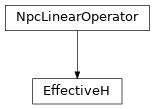# EffectiveH¶

Inheritance DiagramMethods

 `EffectiveH.__init__`(env, i0[, combine, ...]) Return the hermitian conjugate of self Combine the legs of theta, such that it fits to how we combined the legs of self. Calculate the action of the operator on a vector vec. Contract self to a matrix. `EffectiveH.update_LP`(env, i[, U]) Equivalent to `env.get_LP(i, store=True)`; optimized for combine. `EffectiveH.update_RP`(env, i[, VH]) Equivalent to `env.get_RP(i, store=True)`; optimized for combine.

Class Attributes and Properties

class tenpy.algorithms.mps_common.EffectiveH(env, i0, combine=False, move_right=True)[source]

Prototype class for local effective Hamiltonians used in sweep algorithms.

As an example, the local effective Hamiltonian for a two-site (DMRG) algorithm looks like:

```|        .---       ---.
|        |    |   |    |
|       LP----H0--H1---RP
|        |    |   |    |
|        .---       ---.
```

where `H0` and `H1` are MPO tensors.

Parameters
• env (`MPOEnvironment`) – Environment for contraction `<psi|H|psi>`.

• i0 (int) – Index of left-most site it acts on.

• combine (bool, optional) – Whether to combine legs into pipes as far as possible. This reduces the overhead of calculating charge combinations in the contractions.

• move_right (bool, optional) – Whether the sweeping algorithm that calls for an EffectiveH is moving to the right.

length

Number of (MPS) sites the effective hamiltonian covers. NB: Class attribute.

Type

int

i0

Index of left-most site it acts on.

Type

int

dtype

The data type of the involved arrays.

Type

np.dtype

N

Contracting self with `as_matrix()` will result in an N`x`N matrix .

Type

int

acts_on

Labels of the state on which self acts. NB: class attribute. Overwritten by normal attribute, if combine.

Type

list of str

combine

Whether to combine legs into pipes as far as possible. This reduces the overhead of calculating charge combinations in the contractions.

Type

bool

move_right

Whether the sweeping algorithm that calls for an EffectiveH is moving to the right.

Type

bool

combine_theta(theta)[source]

Combine the legs of theta, such that it fits to how we combined the legs of self.

Parameters

theta (`Array`) – Wave function to apply the effective Hamiltonian to, with uncombined legs.

Returns

theta – Wave function with labels as given by self.acts_on.

Return type

`Array`

update_LP(env, i, U=None)[source]

Equivalent to `env.get_LP(i, store=True)`; optimized for combine.

Parameters
update_RP(env, i, VH=None)[source]

Equivalent to `env.get_RP(i, store=True)`; optimized for combine.

Parameters

Return the hermitian conjugate of self

If self is hermitian, subclasses can choose to implement this to define the adjoint operator of self.

matvec(vec)[source]

Calculate the action of the operator on a vector vec.

Note that we don’t require vec to be one-dimensional. However, for square operators we require that the result of matvec has the same legs (in the same order) as vec such that they can be added. Note that this excludes a non-trivial qtotal for square operators.

to_matrix()[source]

Contract self to a matrix.

If self represents an operator with very small shape, e.g. because the MPS bond dimension is very small, an algorithm might choose to contract self to a single tensor.

Returns

matrix – Contraction of the represented operator.

Return type

`Array`